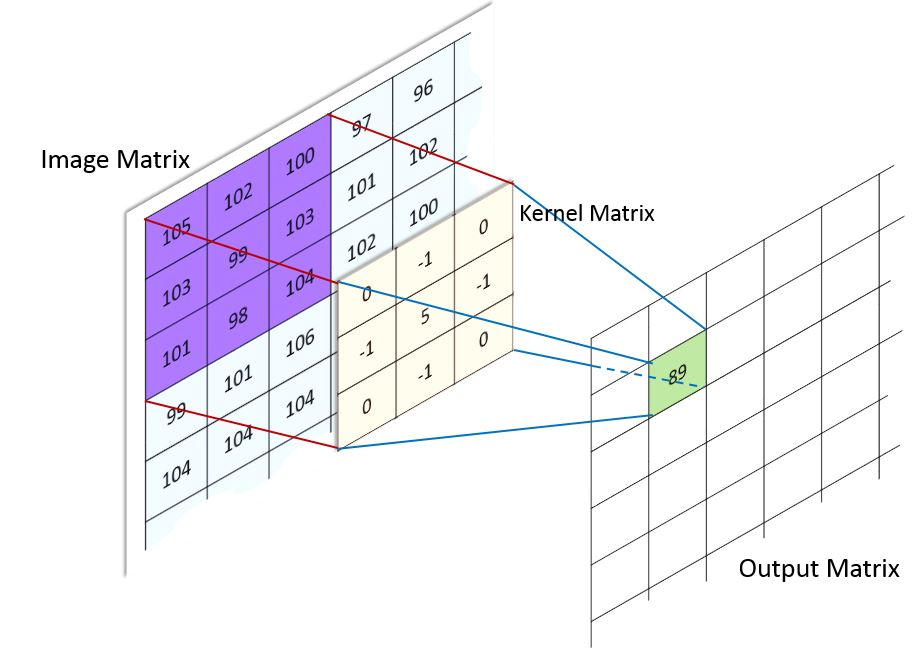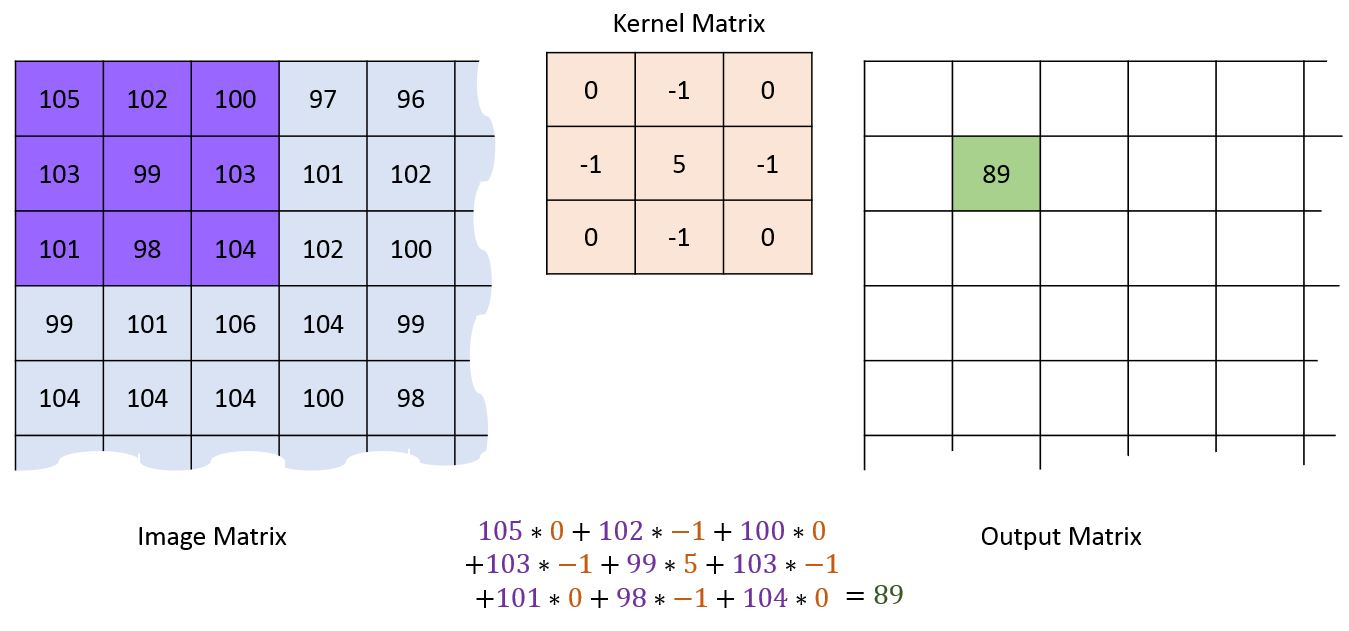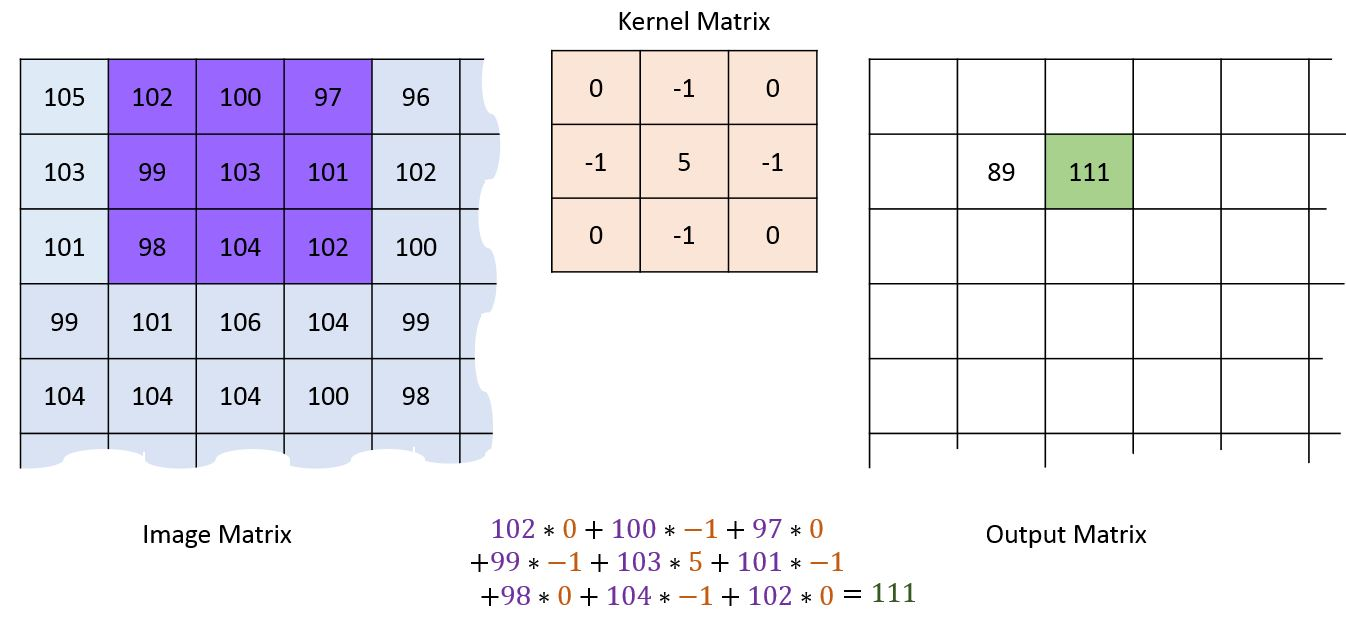# EOJ Monthly 2018.8

A. A Simple Convolution

Convolution is the process of adding each element of the image to its local neighbors, weighted by the kernel. You can skip the following description if you know what that is; if you don’t, there is a brief introduction here.

Images can be thought of as a matrix. Usually, they have three channels: red, green and blue; but here we only consider the case where there is only one channel.

Each convolution operation has a kernel which could be a any matrix smaller than the original image in height and width. Each kernel is useful for a spesific task, such as sharpening, blurring, edge detection, and more. Let’s start with the sharpening kernel which is defined as:

$$K = \begin{bmatrix} 0 & -1 & 0 \ -1 & 5 & -1 \ 0 & -1 & 0 \ \end{bmatrix}$$

Then we do the following steps:

1. Put the first element of the kernel at every pixel of the image (element of the image matrix). Then each element of the kernel will stand on top of an element of the image matrix.1. Multiply each element of the kernel with its corresponding element of the image matrix (the one which is overlapped with it)
2. Sum up all product outputs and put the result at the same position in the output matrix as the center of kernel in image matrix.For the pixels on the border of image matrix, some elements of the kernel might stands out of the image matrix and therefore does not have any corresponding element from the image matrix. For this problem, you can eliminate the convolution operation for these position which end up an output matrix smaller than the input (image matrix).

The problem statement above is copied from here.

### 输入格式

The first line contains two space-separated integers $n$ and $m$ ($1 \le n, m \le 100$).

The next $n$ lines each contains $m$ space-separated integers denoting the image matrix. The range of elements in the image matrix is between $0$ and $255$, inclusive.

The next line contains two space-separated integers $h$ and $w$ ($1 \le h, w \le 100$, $h \le n$, $w \le m$).

The following $h$ lines each contains $w$ space-separated integers denoting the kernel matrix. The range of these elements is $[-128,127]$.

### 输出格式

Output the result of convolution. It should be a matrix of size $(n-h+1) \times (m-w+1)$.

### 样例

Input
5 5
105 102 100 97 96
103 99 103 101 102
101 98 104 102 100
99 101 106 104 99
104 104 104 100 98
3 3
0 -1 0
-1 5 -1
0 -1 0

Output
89 111 101
85 111 101
98 117 113

Input
2 3
1 1 2
3 5 8
2 3
13 21 34
55 89 -122

Output
-264

Input
3 2
0 1
2 3
4 5
1 2
-1 127

Output
127
379
631


NaN